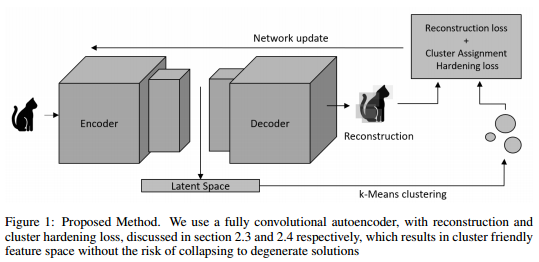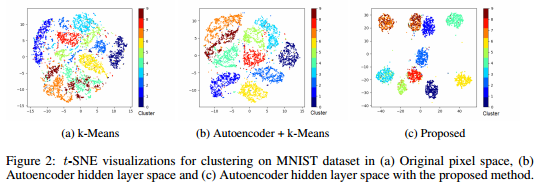NOTE : This paper is more of a review of the current state of clustering using deep learning. So do not expect flashy results.

The authors decompose their problem in 4 sections :

• The architecture
• The number of features to use (1 layer versus multiple)
• The non-clustering loss
• The clustering loss

The first 3 are straigthfoward, it seems that everyone uses a CNN autoencoder.

The last one is interesting because it forces the network to learn features that would be easy to clusters. Given a set of K centers $$\mu$$, a batch of N samples $$z$$ and an association matrix $$s$$, we define the k-means loss as :

$L(\theta)=\sum^{N,K}_{i=1,k=1}s_{i,k} \Vert z_{i}-\mu_{k}\Vert^2$

## Soft assignments

The previous equation has a major issue, it requires hard assignments. The authors show how to compute soft assignments. The authors define $$q_{ij}$$ the similarity between a point $$i$$ and a cluster $$j$$. All points are then made stricter by creationg a distribution P :

$p_{ij} = \frac{\frac{q^2_{ij}}{\sum_{i'}q_{i'j}}}{\sum_{j'}(\frac{q^2_{ij'}}{\sum_{i'}q_{i'j'}})}$

The added loss is the KL divergence between P and Q. This loss forces the network to compute assignments with confidence. The authors also argue that this loss prevent large clusters from distorting the hidden feature space.The authors use a two-step training regime. They first train the autoencoder without the clustering loss and then add it.

The authors show a t-sne plot of their embedding which seems superior to the autoencoder alone.They also test with COIL20 which is a dataset similar to CIFAR10 but with less samples and 20 classes.

CODE : Theano